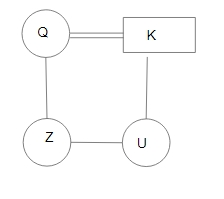Question 11

# $$A - B$$ means ‘A is the mother of B’;$$A \times B$$ means ‘A is the sister of B’:$$A \div B$$ means ‘A is the daughter of B’.Which of the following expressions means 'U is the daughter of Q'?

Solution

From the Option C, 'U' is the daughter of 'Q'.
In the diagram, Circle shows the female, square shows the male, vertical lines show the generation, single horizontal line shows the brother/sister and double horizontal lines show the couple.$$\therefore$$ The correct answer is option B.